# How Do You Know if Your Data Has Signal?Image by Liz Sullivan, Creative Commons. Source: Wikimedia

An all too common approach to modeling in data science is to throw all possible variables at a modeling procedure and “let the algorithm sort it out.” This is tempting when you are not sure what are the true causes or predictors of the phenomenon you are interested in, but it presents dangers, too. Very wide data sets are computationally difficult for some modeling procedures; and more importantly, they can lead to overfit models that generalize poorly on new data. In extreme cases, wide data can fool modeling procedures into finding models that look good on training data, even when that data has no signal. We showed some examples of this previously in our “Bad Bayes” blog post.

In this latest “Statistics as it should be” article, we will look at a heuristic to help determine which of your input variables have signal.

Thought experiment: What does a pure noise variable look like?

To recognize if a variable is predictive of the outcome you are interested in, it helps to know what it looks like when a variable is completely independent of the outcome. Suppose you have a variable `x` that you believe predicts an outcome `y` — in our examples, `y` will be a binary outcome, TRUE or FALSE, but the idea works for general `x` and `y`.

```x1   y1
x2   y2
x3   y3 …
```

We can make `y` independent of `x` by scrambling (or permuting) the order of x and y relative to each other:

```x1  y4
x2  y7
x3  y1 …
```

Now fit a one variable model (say a logistic regression model) to this new no-signal data set, and compare the model’s training performance (say its deviance) to the training performance of a logistic regression model fit to the true data (`x`, `y`). If `x` truly has signal (as you hope), then the model on the unpermuted data should perform much better (have lower deviance) than the model fit to the no-signal (permuted) data. If `x` actually has no signal, then both models should look about the same.

Now do the permutation step over and over again. You will have built up a distribution of models built on no-signal data sets that “look like” the original data — that is, the data sets are the same size as the original data, and they have the same distributions of `x` values and of `y` values as your original data, just not in the same pairings. You also have the distribution of how they perform. If `x` truly has signal, then the model built on the real (`x`, `y`) should perform much better than the other models — its deviance should be to the left (lower) than the “hump” of deviances of the other models. If `x` does not predict `y`, then its deviance will probably sit somewhere within the range of deviances of the other models.

Let’s see what this looks like in R.

```library(ggplot2)
# return a frame of the deviance scores on the permuted data
permutation_test = function(dataf, ycol, nperm) {
nrows = dim(dataf)
y = dataf[[ycol]]
X = dataf[, setdiff(colnames(dataf), ycol), drop=FALSE]
varnames = colnames(X)
fmla = paste("y", paste(varnames, collapse=" + "), sep=" ~ ")
deviances = deviances from perm test
num = sum(vperm <= vdev)
vscore = num/nperm
print(ggplot(data.frame(nullperm=vperm), aes(x=nullperm)) +
geom_density() + geom_vline(xintercept=vdev, color='red') +
ggtitle(paste(title, "left tail area ~", vscore)))

```

Now we build a small data set with one predictive variable and one noise variable, and compare the performance of the two variables.

```set.seed(3266)
N = 1000
s1 = rnorm(N)
n1 = rnorm(N)
y = 2*s1 + rnorm(N)
dframe = data.frame(y=y>0, s1=s1, n1=n1)

nperm=500

# First, the model on the signaling variable
score_variable(dframe, "y", "s1", nperm,
title='Signal variable deviance,')
```

The one-variable model built from the variable with signal has a deviance far smaller than its companion no-signal data sets.```score_variable(dframe, "y", "n1", nperm,
title='Noise variable deviance,')
```

The one-variable model built from the no-signal variable has a deviance that sits right in the middle of the distribution of no-signal data sets: about 36% of the permuted data sets produced models with training deviances lower than the model on the real data. So we have plausible evidence that this variable does not provide any signal about the outcome, and therefore isn’t useful.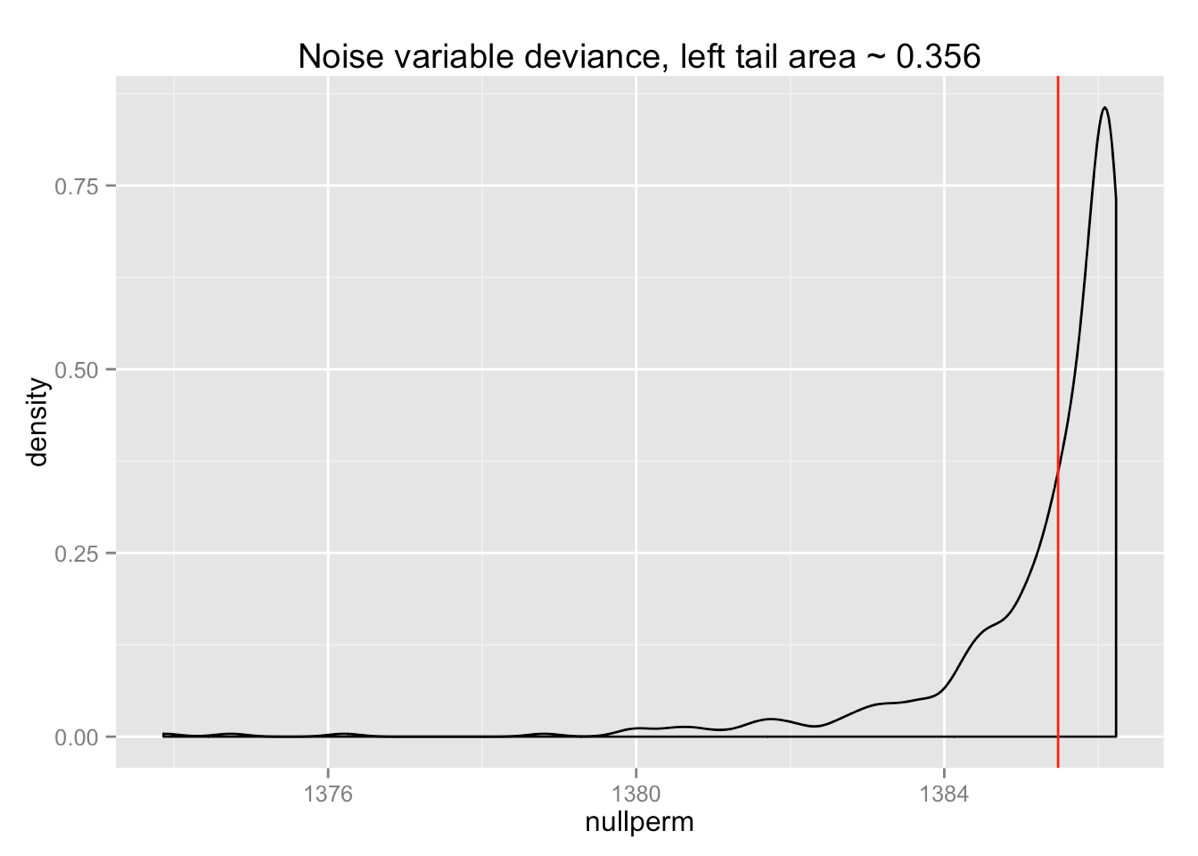This is what “no signal” looks like: almost usable.

This permutation test technique can be used not just for deviance, but for any metric, like accuracy, precision or recall (for classifiers), or squared error (for regression on continuous-valued outcomes). You can even, in principle, use permutation tests to evaluate whether an entire model — not just a single variable — is extracting useful signal from the data. The idea is the same: if your model’s accuracy, or variance, or whatever, falls within the distribution of the performance of models built on permuted (no-signal) data, then the original model is not extracting meaningful, generalizable concepts from the data. The permutation test, in this situation, is directly measuring if your model is statistically significantly different from a family of uninformative models. For shorthand, we will call this “the significance” of the model. Note that we want the significance value (the area under the left tail in the figures above) to be small.

There is a caveat here: this technique won’t work on modeling algorithms that memorize their training data, like random forest (Kohavi noticed the same problem with cross-validation and bootstrap). Random forests regularly fit to perfect accuracy on training data, no matter what, so there isn’t a meaningful distribution to compare against (although one can evaluate a random forest model with a permutation test of deviance). In any case, one doesn’t usually have to worry about calculating the significance of a full model in a data science (data-rich) situation: if you want to determine if a full model is overfitting its training data, it’s better to do that with hold-out data. So we will stick to discussing variable evaluation.

From thought experiment to practical suggestion: chi-squared and F tests

When you have very many variables, permutation tests to check which of the variables have signal can get computationally-intensive. Fortunately, there are “closed-form” statistics you can use to estimate the significance of your variables (or to be precise, the significance of the one-variable models built from your variables). Let’s stick to the example of predicting a binary outcome using logistic regression. You can determine the significance of a logistic regression model by looking at the difference between the model’s deviance on the training data, and the null deviance (the deviance of the best constant model: the mean of `y`). In R parlance, if your glm model is called `model`, then you want to look at `delta_deviance = model\$null.deviance - model\$deviance`. If there is no signal in the data, then this quantity is distributed as a chi-squared distribution with degrees of freedom equal to the difference of the degrees of freedom of each model (`delta_deviance` will have one degree of freedom for every numerical input to the model, and `k-1` degrees of freedom for every k-level categorical variable). The area under the right tail of this chi-squared distribution is the probability that no-signal data would produce `delta_deviance` as large as what you observed. This is the significance value of `model`.

```# get the significance of glm model
get_significance = function(model) {
delta_deviance = model\$null.deviance - model\$deviance
df = model\$df.null - model\$df.residual
sig = pchisq(delta_deviance, df, lower.tail=FALSE)
}
```

For the example that we used above, the signal variable had a chi-squared significance of 1.9e-163, compared to 0 estimated from the permutation test; the no-signal variable had a chi-squared significance of 0.386, compared to 0.356 estimated from the permutation test.

For linear regression, there is a similar statistic called the F-statistic to determine model significance; in R, both the F-statistic and the corresponding model significance (called its p-value) are given by in the summary of a linear regression (`lm()`) model.

So we can heuristically determine which variables have signal for a classification model by looking for variables with small significance value (as estimated by the chi-squared distribution), and for a regression model by looking for variables with small significance value (as estimated by the F distribution). We define “small” by picking a threshold, and accepting variables whose significance value is smaller than that threshold. Most modeling algorithms can handle the presence of a few noise variables, so it’s better to pick a somewhat high threshold to err on the side of accepting useless variables, rather than losing useful ones.

Let’s look at a small example. We’ll generate a dataframe of 1000 rows, with ten input variables: five with signal (g-variables), called `gx_x`, and five without (n-variables), called `nx_x`. The variables are either continuous-valued (`gn_x` or `nn_x`) variables with expected mean zero and unit standard deviation; or categorical variables with three levels (`gc_x\$a`, `gc_x\$b`, and so on), uniformly distributed. The g-variables are additively related to the outcome, with random coefficients. The outcome `y` is again binary. We’ll use a threshold of 0.05. The code to generate the data set and score the variables can be found here.

The graph below shows the variable scores (significances). The threshold is shown as the dashed red line; the variables that fell below the threshold are shown in green.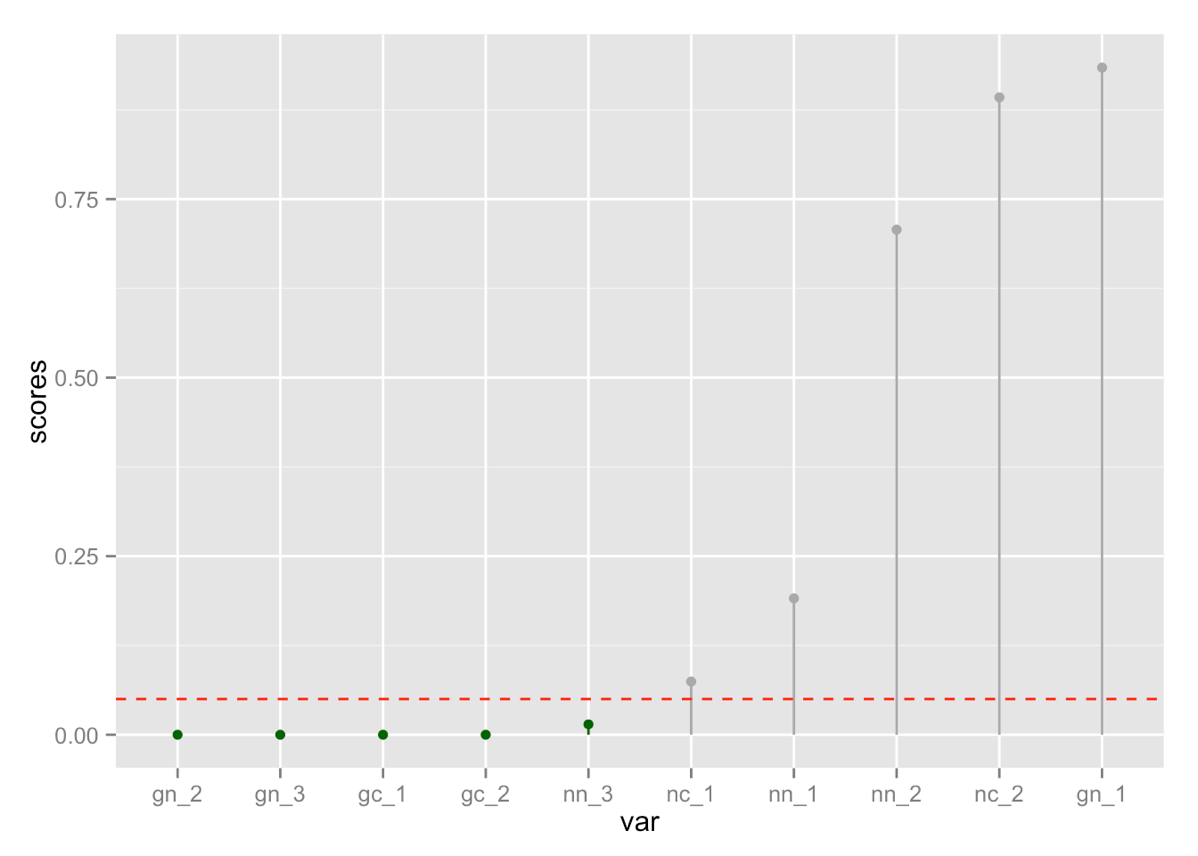```##  "Variables selected:"
##    var       scores
## 2 gn_2 3.909215e-99
## 3 gn_3 3.561903e-09
## 4 gc_1 3.906063e-06
## 5 gc_2 4.325259e-06
## 8 nn_3 1.459305e-02

##  "True coefficients of signal variables"
print(coefs)
## \$gn_1
##  0.03406483
##
## \$gn_2
##  -1.457936
##
## \$gn_3
##  0.4138757
##
## \$gc_1
## \$gc_1\$a
##  -0.504306
##
## \$gc_1\$b
##  -0.2303952
##
## \$gc_1\$c
##  -1.174718
##
##
## \$gc_2
## \$gc_2\$a
##  -0.2770224
##
## \$gc_2\$b
##  -0.1947211
##
## \$gc_2\$c
##  0.2442891
```

In this example we picked four of the g-variables and one of the n-variables. As you can see from the coefficients above, the g-variable that we missed, `gn_1` had a very small magnitude, smaller even than the noise variables (which essentially have magnitude 1), so its signal was weak.

Picking the Threshold

We’ll use a larger example to illustrate picking the threshold. This data set has five signal variables and 2000 noise variables, with 2500 rows. We’ll consider three thresholds: 0.01 (or 1/100), 0.025 (or 1/40) and 0.05 (or 1/20). This time, all the g-variables have appreciable coefficients except `gn_2`.

```## \$gn_1
##  0.8907755
##
## \$gn_2
##  0.09630513
##
## \$gn_3
##  1.072262
##
## \$gc_1
## \$gc_1\$a
##  1.371897
##
## \$gc_1\$b
##  -0.8606408
##
## \$gc_1\$c
##  -0.4705882
##
##
## \$gc_2
## \$gc_2\$a
##  -2.172888
##
## \$gc_2\$b
##  -0.9766713
##
## \$gc_2\$c
##  0.9012387
```

Here are the counts of variables selected by each threshold, along with the variables selected by the strictest threshold, 0.01:

```##  "Variables selected, threshold = 0.01"
##         var        scores
## 1      gn_1  4.744091e-40
## 3      gn_3  4.426174e-72
## 4      gc_1  3.754517e-64
## 5      gc_2 2.838399e-117
## 95    nn_90  7.278843e-03
## 353  nn_348  2.702906e-03
## 470  nn_465  3.625489e-03
## 617  nn_612  8.157911e-03
## 833  nn_828  7.904690e-03
## 1006   nc_1  2.983575e-03
## 1265 nc_260  6.512229e-03
## 1290 nc_285  9.559572e-03
## 1370 nc_365  5.091275e-03
## 1490 nc_485  7.549149e-03
## 1606 nc_601  2.689314e-03
## 1650 nc_645  5.622456e-03
## 1912 nc_907  9.193532e-03
```

The threshold you select indicates how much error you are willing to tolerate — you can think of it as the false positive rate. A threshold of 0.01 is a 1 in 100 false positive rate, so you would expect from 2000 noise variables to select around 20. In this case, we got lucky and selected only 13. A threshold of 0.025 is 1 in 40, so from 2000 noise variables we’d expect around 50 false positives; we picked 42. A threshold of 0.05 is 1 in 20, so from 2000 noise variables we expect about 100 false positives; in this case, we got 98.

This indicates that when you are winnowing down a very large number of variables, if you expect that most of them are noise, then you want to use a stricter threshold.

We can also try fitting a random forest model to this example, once with all the variables, and once with the 17 variables selected by the threshold 0.01. Both models get perfect performance on training. The full model got an AUC of 0.87 on holdout data; the reduced model got an AUC of 0.93. This is clearly a somewhat labored example — you don’t want to fit a model with almost as many columns as there are datums — but it does demonstrate that variable filtering can improve the model. You can see the code for the example here (bottom of the page).

1. Model significance is an indication of the presence of a signal, not the utility of the variable. Just because a variable passes the test doesn’t mean that it is a good or useful one. There is generally a relationship between apparent effect size (strength of signal) and the significance, but the actual predictive value of a variable is for the modeling algorithm to determine. The purpose of this heuristic is to winnow down the obviously useless variables.

2. Use the model significance to threshold the variables, not to sort them. This follows from point 1. Just because variable 1 has a smaller significance value than variable 2 does not mean that variable 1 is more useful than variable 2.

3. This heuristic is based on logistic and linear models, but it isn’t restricted to logistic/linear regression modeling. Once you’ve selected the variables, you can use any modeling procedure to fit a model: random forest, gradient boosting, SVM, whatever you like. The primary assumption of this heuristic is that a useful variable has a signal that could be detected by a linear or logistic model, which seems like a reasonable supposition.

4. Because you are scoring each variable by treating it as an input to a one variable linear/logistic model, you need to take care when handling variables that are in reality the output of a submodel for the outcome. An example is using impact coding to manage categorical variables with a very large number of levels. Impact coding builds a bayesian model for the outcome from a single categorical variable, then uses the output of that submodel as a numerical input to the larger model, instead of the original variable. Because the impact-coded variable is now numerical, it will appear to have one degree of freedom. This is true only if the impact-coded model was built from data distinct from the data you are using to score the variables. If you use the same data to build the impact coding as you are using to score the variables, then the impact coding can potentially memorize the scoring data; this means the impact-coded model in reality has more than one degree of freedom (k-1, where k is the number of levels of the original variable). If you build the impact-coding without looking at the scoring data, then you can’t memorize the scoring data, so it’s safe to assume the impact-coded variable has only one degree of freedom.

From this it follows that if you are going to impact-code, or otherwise build submodels that also predict the outcome from your variables, the submodels should be fit from a separate calibration dataset, not the training set that you will use to score the variables and to fit the full model. This is a good idea not just because of the variable scoring, but because using the same data to fit the submodels and the primary model can introduce undesirable bias into the primary model fitting. See the note at the end of this post (and this post) for more discussion.

1. No automatic variable selection approach is a substitute for domain knowledge; think of this as a tool to help focus your attention on where the information you need might be located.

A More Realistic Example

In the above examples, we had good variables with reasonably strong signals and noise variables with no signal at all. In reality, there will additionally be variables with signal so weak as to be useless — but signal. Depending on the threshold you pick such variables can still have acceptably small significance values. Is this heuristic still useful in those situations?

We tried this approach on the 2009 KDD Cup dataset: data from about 50,000 credit card accounts. Our goal is to predict churn (account cancellation). The raw data consists of 234 anonymized inputs, both numerical and categorical. Many of the variables are sparsely populated, and there were a few categorical variables with a large number of levels. We used our vtreat package to clean the data, in particular to deal with NAs and large categoricals. This inflated the number of input columns to 448, all numerical and all clean. As recommended in point 4 above, we split the data into three sets: one for data treatment (vtreat), one for model fitting, and one for test. You can see the code for this experiment here.

For comparison, here is the performance (ROC curve and AUC) of a logistic regression model built on all 448 variables (AUC=0.69 on test compared to 0.74 on training):And here is the performance of a gradient boosting model also built on all 448 variables (AUC=0.71 on test compared to 0.72 on training):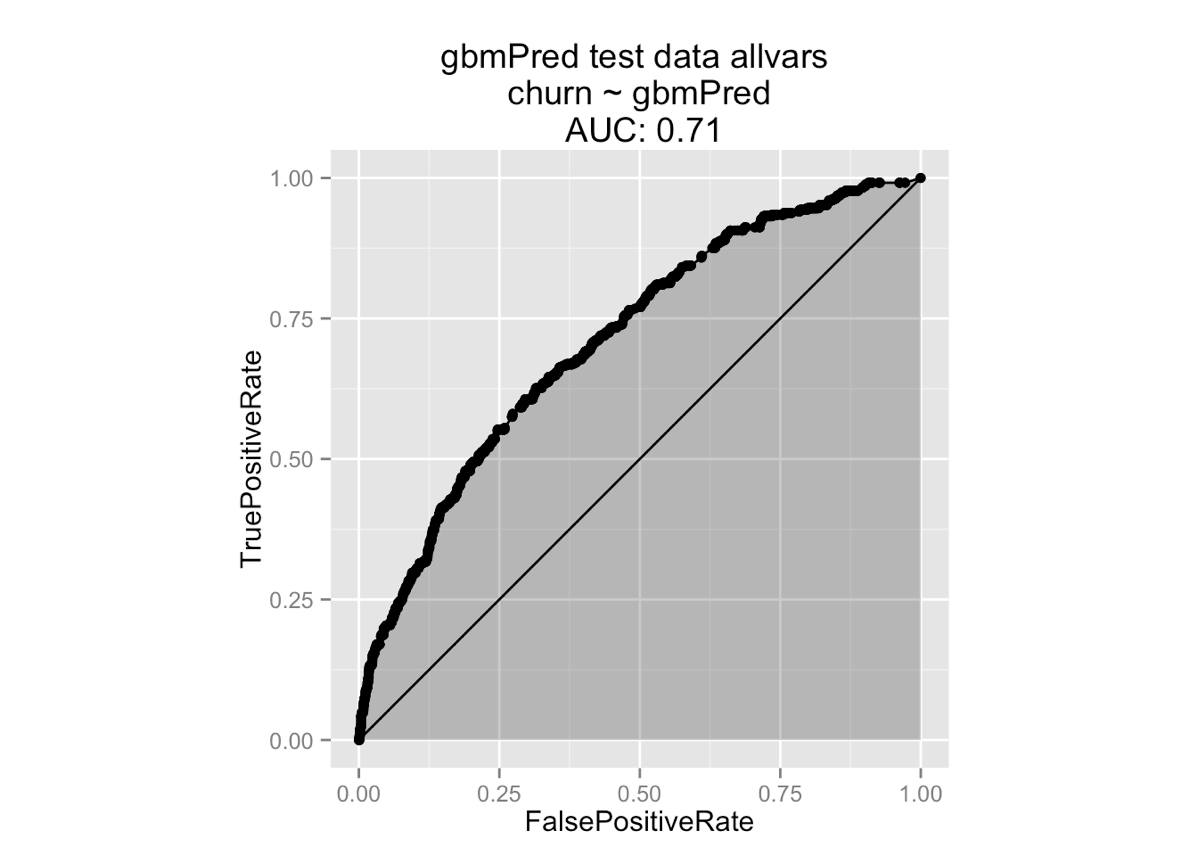Here’s the distribution of variable scores, on a logarithmic (base 10) scale: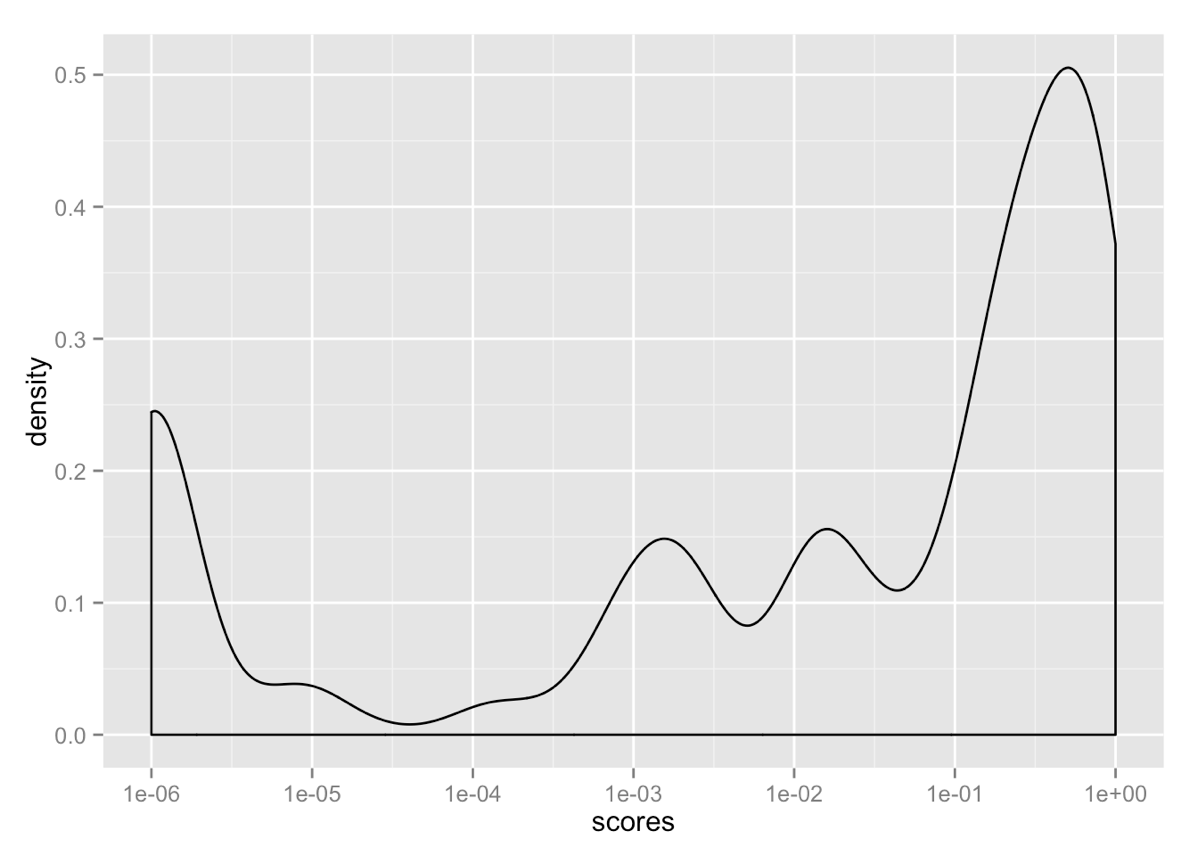The shape of the distribution suggests a mixture of different types of variables, with varying signal strengths. The rightmost population (score greater than 0.05 or so) likely have no signal; the next few populations potentially have a low signal. The shape of the graph suggests that about 3e-5 is a natural cutoff; we loosened this a bit and used a threshold of 10e-4. This reduced the number of variables down to 87. Here are a few of them:

```##   "Var6_isBAD"
##   "Var7_clean"
##   "Var13_clean"
##  "Var65_clean"
##  "Var72_clean"
##  "Var73_clean"
...
##  "Var218_lev_x.cJvF"
##  "Var218_lev_x.UYBR"
##  "Var218_catB"
##  "Var221_lev_x.d0EEeJi"
##  "Var221_lev_x.oslk"
```

The `_clean` variables are numeric (with bad values like NA and `Inf` converted to zeros); the `isBAD` variables are indicator variables created by vtreat to mark unpopulated or otherwise NA fields in the corresponding variable. The `catB` variables are impact-coded, and the `_lev_` variables are indicator variables for specific levels of the corresponding categorical variable.

What is interesting is the number of `isBAD` variables (without their corresponding `clean` component) in the final set. This indicates that for these variables the signal is contained in whether or not the field was populated, rather than in the actual value.

Here’s logistic regression’s performance on the reduced variable set (AUC=0.71 on test vs. 0.73 in training):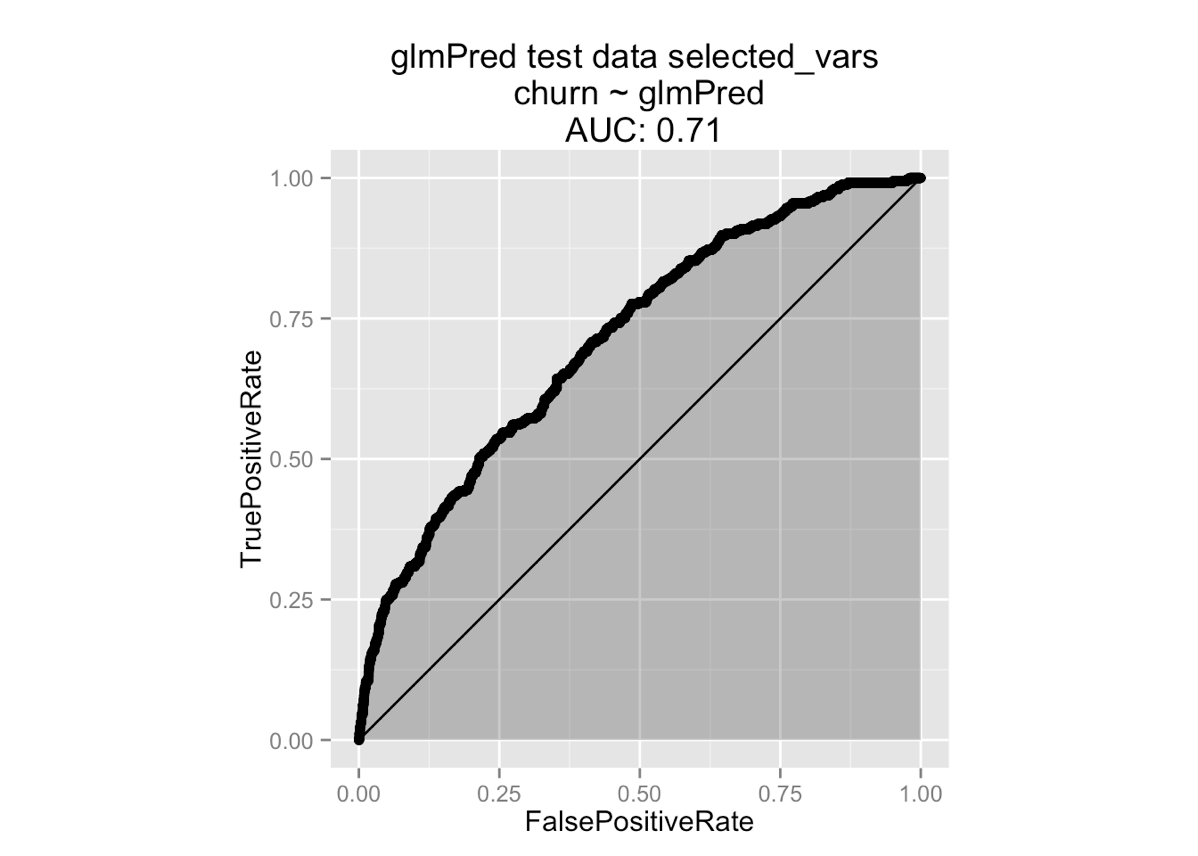And gradient boosting (AUC=0.71 in both training and test).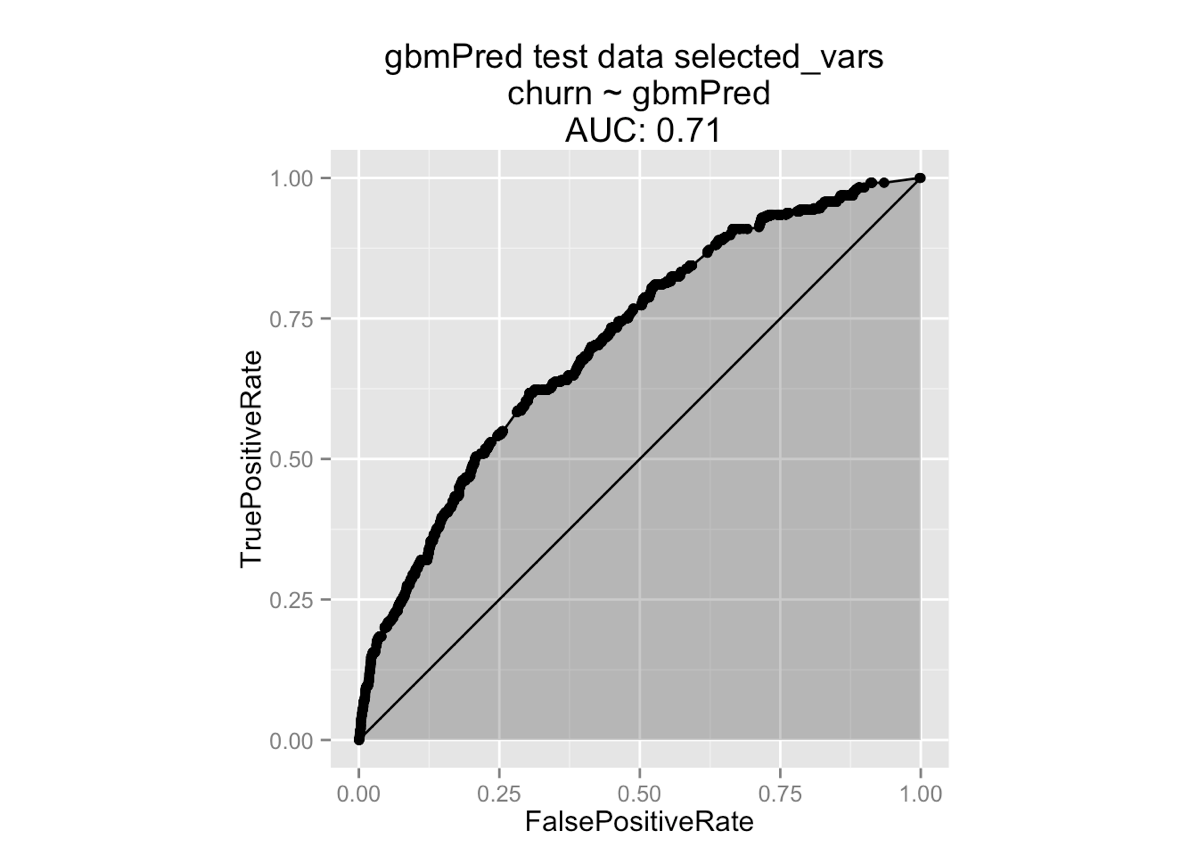Results

Winnowing down the variables didn’t improve model performance much for logistic regression, and not at all for gradient boosting, which suggests that the gradient boosting algorithm does a pretty good job of variable selection on its own. However, the variable filtering reduced the run time for gradient boosting by almost a factor of five (from 7 seconds to 1.5), and that in itself is of value.

Takeaways

We’ve demonstrated a heuristic for determining whether or not an input variable has signal. We derived our heuristic by empirical exploration (the permutation test), and then noticed that there is an existing standard statistical test (the chi-squared test for logistic regression model significance) that gives us the measure that we want. This is a good general practice: pick your statistical test based on what you want to measure, or what you are trying to defend against, and only then settle on a procedure.

You can find the code for these examples as R markdown, along with html of the runs, here.### nzumel

Data scientist with Win Vector LLC. I also dance, read ghost stories and folklore, and sometimes blog about it all.

### 9 replies ›

1.Scott Locklin says:

“This indicates that for these variables the signal is contained in whether or not the field was populated, rather than in the actual value.”

For whatever reasons, I find this is very often the case with industrial data. There is probably some deep philosophical thing here, but is.na has become my go-to feature generation gizmo.

1.Nina Zumel says:

We’ve found this to be the case, as well. It’s a practitioner vs. theorist thing, I think. In practice, NAs are there for a reason — often an interesting one — but much of the stat literature on missing variables wants to treat them as randomly missing data (“faulty sensor”), and impute values for them, which misses the point.

2.John R. says:

Speaking of impact coding, I can’t find any listing of vtreat on CRAN. Is the push to get that published still moving forward?

1.jmount says:

Thanks for the kind question!

We cleaned up the package quite a bit, but want to firm up the variable scoring interface before submitting to CRAN. Right now vtreat uses PRESS style scoring to get an unbiased estimate of out of training performance as an effect size (even for categorical variables). Adding a significance (like a permutation test) is tempting. And honestly the convenience of `devtools::install_github("WinVector/vtreat")` slowed us down a bit.

Long story short- we are still working on getting ready to submit to CRAN. It is up! And we were able to re-work a few of Nina’s examples here.

3.Stephen says:

I first saw this trick during a talk by Phil Briely an Australian based Data Miner who came first in the Heritage Health Prize a few years ago. He mentions the idea in this talk. https://youtu.be/1fIyQL9FiAk

1.jmount says:

An interesting reference on the topic is “Permutation Tests”, 2nd edition, Philip Good, Springer 2000.

4.nowgis says:

Thanks for a great article.

5.Nicolás says:

Thanks for the article!! Just a small precisión: in a linear regression, individual test over variables is not a F test but a t test. F test is the inference about the joint validity of all explanatory variables.

1.jmount says:

Actually, I think she knew of both tests and in fact applied the correct one.

In the article Nina is checking the significance of the quality of each single-variable model fit separately (under a null hypothesis). This has one asking questions about non-negative quantities (ratios of variances) and hence the F-test. Each time a model is fit using only one variable (and the dc-term), so with the right degrees of freedom it is an F-test of all “one variables” jointly.

The t-test could be used to check the significance of coefficient difference from zero (under a null hypothesis), which is an interesting but different question. And yes, when fitting many coefficients in one model you can quickly apply the t-test to get coefficient significances (and could use that to filter variables), but that isn’t what Nina happened to describe.

Now if you were to look at the quality of the best single variable model you would have to make some multiple experiment correction, but that is always the case.